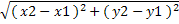A | B | C | D | E | F | G | H | I | J | K | L | M | N | O | P | Q | R | S | T | U | V | W | X | Y | Z

# Mathematics-Euclidean Distance

It is the distance between the 2 points in the cartesian coordinates ( x1, y1 ) and ( x2, y2 )

So Euclidean distance, d is calculated as =This is called Pythagora's theorem in Cartesian system